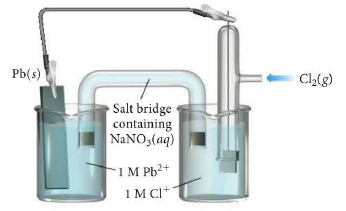# Problem: Consider the voltaic cell: a. Determine the direction of electron flow and label the anode and the cathode. b. Write a balanced equation for the overall reaction and calculate E°cell. c. Label each electrode as negative or positive.  d. Indicate the direction of anion and cation flow in the salt bridge.

###### FREE Expert Solution
88% (286 ratings)
###### Problem Details

Consider the voltaic cell:

a. Determine the direction of electron flow and label the anode and the cathode.

b. Write a balanced equation for the overall reaction and calculate E°cell.

c. Label each electrode as negative or positive.

d. Indicate the direction of anion and cation flow in the salt bridge.What scientific concept do you need to know in order to solve this problem?

Our tutors have indicated that to solve this problem you will need to apply the Galvanic Cell concept. You can view video lessons to learn Galvanic Cell. Or if you need more Galvanic Cell practice, you can also practice Galvanic Cell practice problems.

What is the difficulty of this problem?

Our tutors rated the difficulty ofConsider the voltaic cell: a. Determine the direction of el...as medium difficulty.

How long does this problem take to solve?

Our expert Chemistry tutor, Jules took 3 minutes and 34 seconds to solve this problem. You can follow their steps in the video explanation above.

What professor is this problem relevant for?

Based on our data, we think this problem is relevant for Professor Statler's class at UTAH.

What textbook is this problem found in?

Our data indicates that this problem or a close variation was asked in Chemistry: A Molecular Approach - Tro 3rd Edition. You can also practice Chemistry: A Molecular Approach - Tro 3rd Edition practice problems.